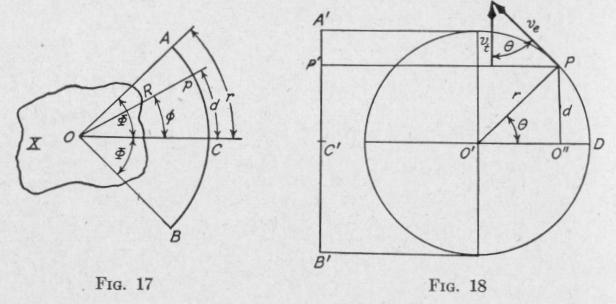Previous Page Index(For best results view this page as a PDF) Next Page

26   PRINCIPLES OF ELEMENTARY DYNAMICS

lar displacement of the body from the equilibrium position is 0. The symbol S represents the torsional stiffness, [L/¢], of the body.

Angular Velocity. - Figure 17 represents a body X that is vibrating with simple harmonic motion of rotation through an amplitude 43 about an axis normal to the plane of the diagram through the point 0. The point p of a line op, fixed in the body, moves back and forth with simple harmonic motion of translation in the arc AB. The distance AB is shown as a straight line A'B'

in Fig. 18. The simple harmonic motion of translation along A'B' is also the motion of the projection P' of a point P that moves with uniform speed in the circumference of a circle of diameter equal to A'B' and in the same period as the simple harmonic motion of rotation of the body X.

Suppose that the point P moves with uniform speed ve around the circle and that in time t after passing the position D, the radius 0'P has moved through an angle 0. At this instant, the component velocity in the direction A'B', that is, the velocity of the projected point P', has the magnitude

V, = ve COS 0

In Fig. 18, the linear velocity of the point P' when at the equilibrium position is ve, and the value at t seconds later when the body X has rotated through an angle 0, is v,. Dividing each member of this equation by the distance R of the point p from the axis of vibration 0,

V1   Ve

R R cos 0
or, (16):   w, = we cos 0   (38)

SIMPLE HARMONIC MOTION   2

where we and w, represent, respectively, the angular velocities of the body X when moving through the equilibrium position, and t seconds later.

In Fig. 18 the angle 0 equals the product of the angular velocity of the line 0'P and the time t occupied in moving through this angle. If time T is occupied in making one entire revolution about 0', then the angular velocity of 0'P has the magnitude 2,r/T. Hence, the angle

and (38) becomes

w, = we COS 2Tt   (40)

In this equation time is reckoned from the equilibrium position. If it be reckoned from one end of the oscillation, that is it/2 radians from the equilibrium position, we would have

ws = We COS ( 2T    t - 2) = We sin 2T t   (41)

The maximum angular displacement from the equilibrium position is called the amplitude of the vibration. Another useful formula for the angular velocity is one involving the period T, angular amplitude 4' and angular displacement 0. From Fig. 18, the linear velocity of a body moving with simple harmonic motion of translation of period T and amplitude r at the instant when the displacement from the equilibrium position is d has the value

0'

v,[= ve cos 0] = 2 T 7rr ( r    0") = 2 T    a,r2 - d2   (42)

From Fig. 17, (7), and (16)

r = 4'R, d = ¢R and v, = w,R   (43)

Substituting these values in (42) we find

w, = T V 4)2 - 02   (44)

At the equilibrium position, 0 = 0. When at this angular displacement, the velocity we has the magnitude, (44):

We = T 43 radians per sec.   (45)Previous Page Index(For best results view this page as a PDF) Next Page